“集智百科精选”是一个长期专栏，持续为大家推送复杂性科学相关的基本概念和资源信息。作为集智俱乐部的开源科学项目，集智百科希望打造复杂性科学领域最全面的百科全书，欢迎对复杂性科学感兴趣、热爱知识整理和分享的朋友加入！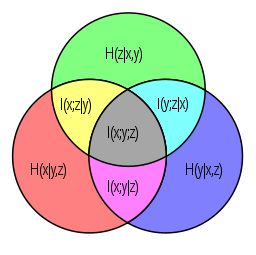对于具有支持集 Probability theory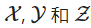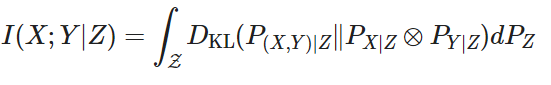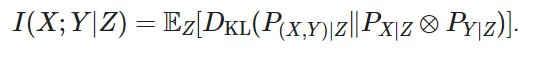对于具有支持集X,Y和Z的离散随机变量条件互信息I(X;Y|Z)如下: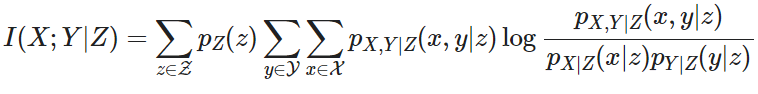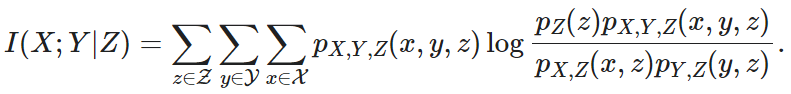对于具有支持集X,Y和Z的（绝对）连续随机变量条件互信息I(X;Y|Z)如下: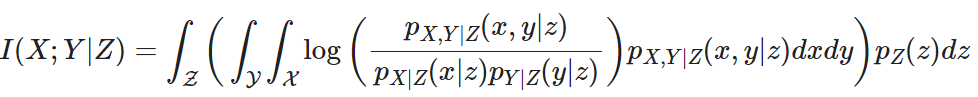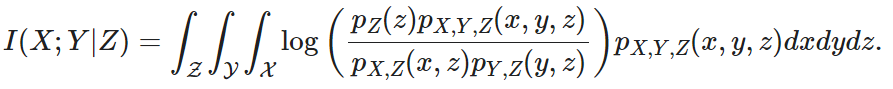同时我们也可以将联合和条件熵写为：I(X;Y|Z) = H(X,Z) + H(Y,Z) – H(X,Y,Z) – H(Z) = H(X|Z) – H()X|Y,Z) = H(X|Z) + H(Y|Z) – H(X,Y|Z)

I(X;Y|Z) = I(X;Z) + I(X;Y|Z)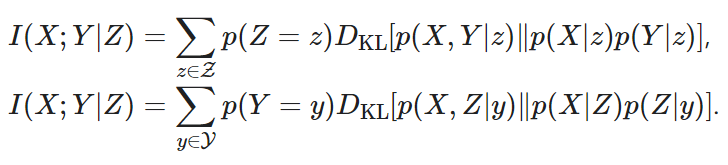条件互信息的其他通用定义（适用于具有连续或其他任意分布的随机变量）将取决于 正则条件概率 Regular conditional probability 的概念。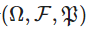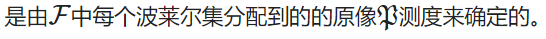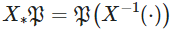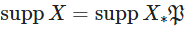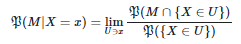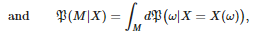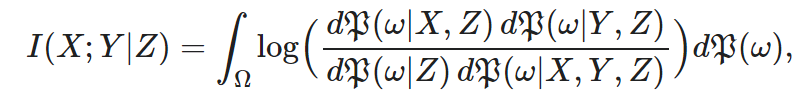非负性

I(X;Y|Z)>=0。

交互信息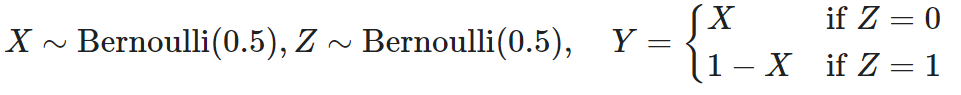X，Y和Z是成对独立的，特别是I(X;Y)=0，不过这里I(X;Y|Z)=1。

互信息的链式法则

I(X;Y,Z)=I(X;Z)+I(X;Y|Z)

结合信息图中的集合或度量理论，可以用条件互信息来归纳定义多元互信息。其定义表达式如下：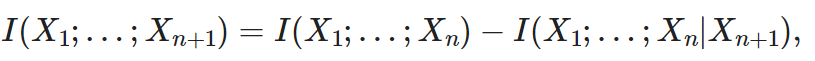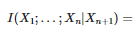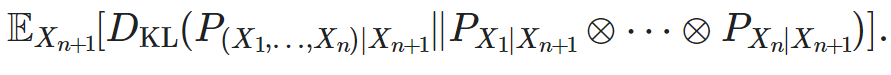集智课程

信息论

https://campus.swarma.org/course/3164

相关文章

• Python 信息熵 条件信息熵 互信息（信息增益）的理解以及计算代码

https://www.pianshen.com/article/465233008/

• R语言互信息和条件互信息

https://blog.csdn.net/tandelin/article/details/104216570